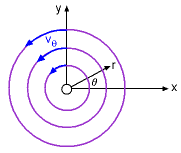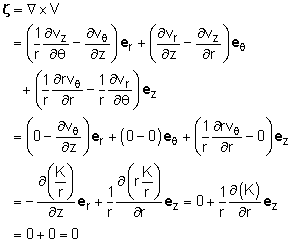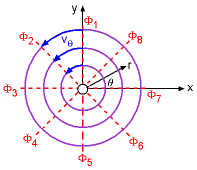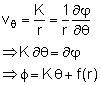Ch 3. Fluid Kinematics Multimedia Engineering Fluids FlowDescriptions Steady &Unsteady Streamlines,Streaklines Velocity &Acceleration IrrotationalFlow
 Chapter 1. Basics 2. Fluid Statics 3. Kinematics 4. Laws (Integral) 5. Laws (Diff.) 6. Modeling/Similitude 7. Inviscid 8. Viscous 9. External Flow 10. Open-Channel Appendix Basic Math Units Basic Equations Water/Air Tables Sections Search eBooks Dynamics Fluids Math Mechanics Statics Thermodynamics Author(s): Chean Chin Ngo Kurt Gramoll ©Kurt GramollFLUID MECHANICS - CASE STUDY SOLUTIONFree Vortex The velocity components of the free vortex are given by      vr = 0   and    vθ = K/r   (assumed vz = 0) The vorticity can be determined by the curl of the velocity as follows:Since the vorticity is zero, Tom has learned that the given free vortex is indeed irrotational.Equipotential Lines The velocity potential associated with the free vortex can then be determined as follows:Since Vr = ∂φ/∂r, the velocity potential is not a function of r, and f(r) reduces to an arbitrary constant. For simplicity, set f(r) = 0 (the value of an individual velocity potential is irrelevant) to yield      φ = Kθ Note that when a flow is referred to as irrotational, it simply implies that the individual fluid elements do not rotate. Although the fluid elements of the given free vortex follow a circular path, it is still irrotational flow.

Practice Homework and Test problems now available in the 'Eng Fluids' mobile app
Includes over 250 problems with complete detailed solutions.
Available now at the Google Play Store and Apple App Store.